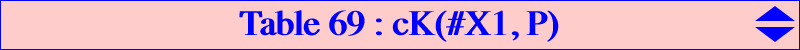A cubic (K) = cK(#X1, P) is an isogonal nodal conico-pivotal cubic. See Special Isocubics §8 for general properties. For any M on (K), its isogonal conjugate M* also lies on (K) and the line MM* (when defined) is tangent to a conic called the pivotal conic (PC) of (K). Here, (PC) is inscribed in the excentral triangle for any P. (K) meets the sidelines of ABC again at U, V, W on the trilinear polar (L) of the root P and then (PC) is also inscribed in the triangle bounded by the lines AU, BV, CW. M and M* are then conjugated with respect to a fixed circle (C) passing through X(1) which is orthogonal to the circles with diameters AU, BV, CW. X(1) is a node on (K) and the nodal tangents are the tangents drawn from X(1) to (PC). Let (I) be the circum-conic with perspector X(1) and center X(9), passing through X(88), X(100), X(162), X(190), X(651), X(653), X(655), X(658), X(660), X(662), X(673), X(771), X(799), X(823), X(897), X(1156), X(1492), X(1821), X(2349), X(2580), X(2581), etc. (K) is acnodal (resp. crunodal) when P is interior (resp. exterior) to (I). When P lies on (I), (K) splits into a line and a circum-conic. *** Special cubics (K) • (K) is equilateral if and only if P = X(4383) and then it is K085. • (K) is a K0 (no term in x y z) if and only if P lies on the antiorthic axis, the trilinear polar of X(1). In this case, it is the X(1)-Hirst inverse of the circum-conic with perspector P and also the X(1)-line conjugate of the bicevian conic C(X1, P*). • (K) is circular if and only if P lies on the trilinear polar of X(7). In this case, the nodal tangents are perpendicular and the singular focus F lies on (K) and on the circumcircle (O). Hence (K) is a strophoid. (PC) is a parabola whose focus is the reflection of X(1) in F and whose directrix is the orthic line of (K) i.e. the parallel at X(1) to the real asymptote of (K). See CL003 for further geometric properties. *** A construcion of (K) (K) is the image of the circum-conic (I) under the transformation 𝛕P which is described in page K1065. With P = u : v : w, this transformation is given by 𝛕P : X = x : y : z → X' = x^2 (w y - v z) : y^2 (u z - w x) : z^2 (v x - u y).The following table gives a selection of these cubics cK(#X1, P). The red point is the singular focus of a strophoid.P cubic X(i) on the cubic for i = type / note 650 K040 1, 105, 243, 296, 518, 1155, 1156, 2651, 2652, 5205, 7061, 9432, 12008, 14189, 14190, 14191, 14192, 14193, 14194, 14195, 14196, 14197, 14198, 14199, 14200, 14201, 14202, 14203, 14204 Strophoid, cK0, note 4 4383 K085 1, 23830, 23831, 23832, 23833, 23834, 23835, 23836, 23837 cK60 514 K086 1, 36, 80, 106, 519, 1323, 1785, 1795, 4845, 5127, 5209, 5526, 5620, 7343 Strophoid 513 K137 1, 44, 88, 239, 241, 292, 294, 1931, 9278 cK0 10015 K165 1, 3, 4, 952, 953, 3109, 6790, 14260, 14887 Strophoid 661 K221 1, 240, 293, 896, 897, 1757, 1758, 1929, 1966, 1967, 2648, 17763, 17954, 17955, 17956, 17957, 17958, 17959, 17960, 18028 cK0 1 K228 1, 1022, 1023 note 1 665 K359 1, 1083, 3110, 5091, 14665, 14839, 14947 Strophoid 14543 K383 1, 2, 3, 4, 6, 3945 see CL061 57 K407 1, 23703, 23704, 23705, 23706, 23838 see CL046 2617 K457 1, 19, 63, 3375, 3376, 3377, 3378, 3383, 3384, 3400, 3401, 3402, 3403, 3404, 3405, 3408, 3409, 21061 101 K588 1, 10, 58, 1794, 1838, 3864, 3865, 4792, 6212, 6213, 10471, 10481, 10482, 10563 6 K635 1, 876, 885, 2283, 3573, 23343, 23352, 23353, 23354, 23355 1633 K689 1, 2, 6, 241, 294, 948, 949, 2303, 6203, 6204, 7347, 7348 see CL061 108 K1073 1, 219, 222, 278, 281, 1172, 1214, 6212, 6213 3732 K1075 1, 3, 4, 218, 277, 1010, 1245, 1780, 3494, 3502, 8481, 8482, 8926, 8947, 8949 652 K1076 1, 1936, 1937, 2635, 2659, 2660, 23691, 23692, 23693, 23694, 23695, 23707 cK0 109 1, 9, 57, 226, 284, 3687, 8072, 8073 649 1, 238, 291, 899, 2107, 2669, 9364, 9365 cK0 664 1, 8, 56, 220, 225, 279, 283, 18798, 18799 1783 1, 3, 4, 28, 72, 6212, 6213, 17170 1983 1, 993, 994, 5692, 5902, 7951, 9275, 15175 3570 1, 2, 6, 31, 75, 1403, 2276, 7155, 14621 4369 1, 740, 741, 5018, 5143, 5524, 7281, 7312, 8481, 8482 Strophoid, note2 4551 1, 9, 57, 3179, 6212, 6213, 7150, 11679, 17185, 18798, 18799 4554 1, 200, 269, 314, 1402, 8947, 8949, 18798, 18799 14838 1, 484, 758, 759, 3065 Strophoid, note 3note 1 : K228 is a circum-conico pivotal cubic. (PC) is the circum-ellipse (I) with perspector X(1) mentioned above. note 2 : the nodal tangents are parallel to the asymptotes of the Kiepert hyperbola. note 3 : the nodal tangents are parallel to the asymptotes of the Jerabek hyperbola. note 4 : the nodal tangents are parallel to the asymptotes of the Feuerbach hyperbola.